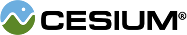#CatmullRomSpline

#### new Cesium.CatmullRomSpline(options) Core/CatmullRomSpline.js 146

A Catmull-Rom spline is a cubic spline where the tangent at control points, except the first and last, are computed using the previous and next control points. Catmull-Rom splines are in the class C1.
Name Type Description
`options` Object Object with the following properties:
Name Type Description
`times` Array.<Number> An array of strictly increasing, unit-less, floating-point times at each point. The values are in no way connected to the clock time. They are the parameterization for the curve.
`points` Array.<Cartesian3> The array of `Cartesian3` control points.
`firstTangent` Cartesian3 optional The tangent of the curve at the first control point. If the tangent is not given, it will be estimated.
`lastTangent` Cartesian3 optional The tangent of the curve at the last control point. If the tangent is not given, it will be estimated.
##### Example:
``````// spline above the earth from Philadelphia to Los Angeles
var spline = new Cesium.CatmullRomSpline({
times : [ 0.0, 1.5, 3.0, 4.5, 6.0 ],
points : [
new Cesium.Cartesian3(1235398.0, -4810983.0, 4146266.0),
new Cesium.Cartesian3(1372574.0, -5345182.0, 4606657.0),
new Cesium.Cartesian3(-757983.0, -5542796.0, 4514323.0),
new Cesium.Cartesian3(-2821260.0, -5248423.0, 4021290.0),
new Cesium.Cartesian3(-2539788.0, -4724797.0, 3620093.0)
]
});

var p0 = spline.evaluate(times[i]);         // equal to positions[i]
var p1 = spline.evaluate(times[i] + delta); // interpolated value when delta < times[i + 1] - times[i]``````

### Members

#### readonlyfirstTangent : Cartesian3 Core/CatmullRomSpline.js 231

The tangent at the first control point.

#### readonlylastTangent : Cartesian3 Core/CatmullRomSpline.js 245

The tangent at the last control point.

#### readonlypoints : Array.<Cartesian3> Core/CatmullRomSpline.js 217

An array of `Cartesian3` control points.

#### readonlytimes : Array.<Number> Core/CatmullRomSpline.js 203

An array of times for the control points.

### Methods

#### evaluate(time, result) → Cartesian3 Core/CatmullRomSpline.js 286

Evaluates the curve at a given time.
Name Type Description
`time` Number The time at which to evaluate the curve.
`result` Cartesian3 optional The object onto which to store the result.
##### Returns:
The modified result parameter or a new instance of the point on the curve at the given time.
##### Throws:
• DeveloperError : time must be in the range `[t0, tn]`, where `t0` is the first element in the array `times` and `tn` is the last element in the array `times`.

#### findTimeInterval(time) → Number Core/CatmullRomSpline.js 273

Finds an index `i` in `times` such that the parameter `time` is in the interval `[times[i], times[i + 1]]`.
Name Type Description
`time` Number The time.
##### Returns:
The index for the element at the start of the interval.
##### Throws:
• DeveloperError : time must be in the range `[t0, tn]`, where `t0` is the first element in the array `times` and `tn` is the last element in the array `times`.